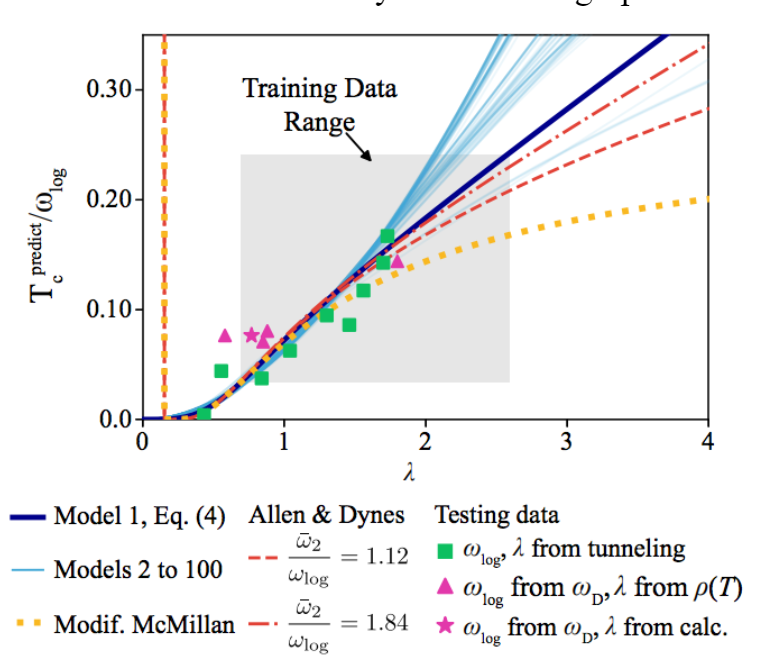top of page
Search

# Using Machine Learning to Derive Critical Temperature Equation

By Fakharyar KhanWhen a current passes through a material, the electrons collide with positive ions which causes small deviations in the electrons’ path which creates a resistance to the flow of electrons. For Ohmic conductors, resistance varies linearly with temperature but when a material reaches a very low temperature, its critical temperature, the resistance abruptly drops to zero and the material becomes a superconductor.

The critical temperature of a superconductor is dependent on a variety of factors such as the conductor’s structure, phonon-electron interaction, Cooper pairs, and Coulomb interactions. The behaviors of these factors themselves are complex and their interactions even more so. For this reason, there haven’t been many successful theoretical predictions of critical temperatures as they can be computationally taxing and occasionally prone to error. In 1957, a group of researchers created the BCS theory which accurately predicts critical temperatures for many superconductors. In BCS theory, an electron in a lattice attracts positive ions which causes a distortion and collective excitation (a phonon) of the lattice. Another electron would then be attracted to this region and this creates a Cooper pair. This in turn will influence other electrons creating a mesh network of Cooper pairs. This network increases the energy required to break a single Cooper pair which means at sufficiently low temperatures, the vibrations of atoms, the electron-phonon interaction, which are responsible for resistance doesn’t affect the electron flow. There have been improvements and generalizations of this theory such as the Eliashberg theory and the Allen-Dynes equation but they have more variables and constants (more computations) than BCS theory.

Researchers at the University of Florida wanted to use machine learning to derive an approximate formula using data on critical temperatures. They used a machine learning algorithm called SISSO which takes in hundreds of features (variables) that are candidates for affecting the output variable, critical temperature. SISSO then expands the feature space by creating combinations of features linked by unary and binary operators until it reaches a certain level. At each level, SISSO minimizes the error of the regression models and the number of non-zero coefficients through Lo regularization. It uses data gathered on several materials to trim down the feature space by ranking them by their correlation magnitude.

Once they trimmed down the set of expressions, they gave them a training set from data on 9 superconductors to model after. However, when you do this, your expressions can become too fit for the training set and model it too well. This can be bad if your training set isn’t representative of the population due to selection bias. So the researchers used a leave-one-out cross- validation which is where they fit the model to n - 1 data points in the training set and then test its fitness by a single data point in the testing set. They then repeat the process with another combination of n-1 data points until they have exhausted all combinations. They take the mean square error of the individual errors and use that to access its fitness. This technique is a special case of the leave-p-out cross-validation which is much more accurate but also is very inefficient for large p so using the former method was in my opinion the best choice.

The optimal equation that their algorithm produced was: When compared to the testing data set, it had a root square mean error of only 0.25K. The graph below shows the Allen-Dynes equation and the McMillan equation compared through the testing data which yielded an RMSE of 0.30K and 0.92K respectively.Although the equation is much more accurate than past predictions, it has some limitations. Its asymptotic behavior is linear which contradicts that of the Allen-Dynes equations which tends towards λ^0.5. For superconductors with higher critical temperature, the equation had an RMSE of 3.2K. However, this is because they had kept the u term constant at 0.1 due to a lack of data. I don’t think the purpose of this paper was to derive an equation for critical temperature but to demonstrate that machine learning can be used to create more accurate formulas for it.

As stated by the researchers, they didn’t have enough data to create a more representative training and testing set which made their equation inaccurate for outliers and superconductors of higher temperature. Limiting the training set to the data of only 9 superconductors made the equation overfit for that data. They should have taken in more data and if the cross fit algorithm would have become too inefficient because of this, they should have used a non-exhaustive techniques like k-fold cross validation. This would increase the chance of having the equation be too fit but when we increase the sample size, we are also reducing the chance of a selection bias so it shouldn’t the accuracy of the equation. I think that the idea of using machine learning to create approximate formulas is very clever but it requires much more data and also doesn’t have the same insight and intuition that theoretical predictions have.

## Sources

arXiv:1905.06780 [cond-mat.supr-con]

https://github.com/rouyang2017/SISSO

“Machine Learning.” GeeksforGeeks, www.geeksforgeeks.org/machine-learning/.

See All
bottom of page